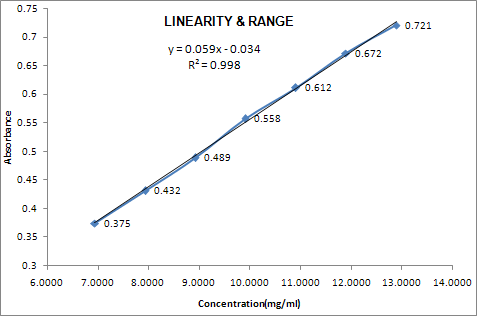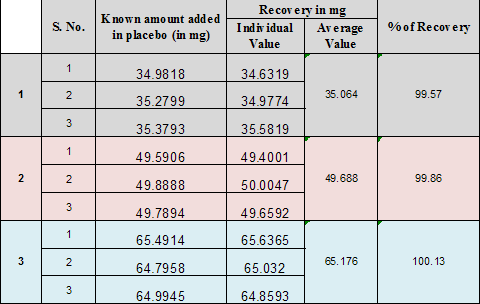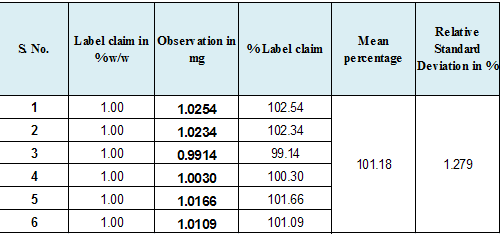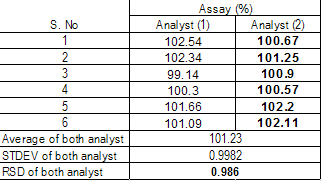# INVOLVEMENT OF HEALTHCARE REGULATORY AFFAIR PROFESSIONAL IN OVERSEAS REGISTRATION PROCESS

Recommended Data:

LINEARITY:
The linearity of an analytical procedure is its ability (within a given range) to obtain test results which are directly proportional to the concentration (amount) of analyte in the sample.

It may be evaluated by visual inspection of a plot of signals as a function of analyte concentration or content. If there is linear relationship, tests results should be evaluated by appropriate statistical method.

Linearity should be evaluated by visual inspection of plot of signals as function of analyte concentration or content. If there is linear relationship, test result should be evaluated by appropriate statistical methods.

Linearity should be determined concurrently during the accuracy study .Classical linearity acceptance criteria are 1) that the correlation coefficient of the linear regression lines is not more than some number close to 1, and 2) that the y- intercept should not differ significantly from zero.

When linear regression analyses are performed, it is important not to force the origin as (0, 0) in the calculation. This practice may significantly skew the actual best-fit slope through the physical range of use.
Visual inspection of standard plot.
Correlation coefficient.
Y-intercept.
Slope.
Residual sum of squares.
Name of Ingredient: (I1): API

RANGE:
The range of an analytical procedure is the interval between the upper and lower concentration (amounts) of analyte in the sample (including these concentrations) for which it has been demonstrated that the analytical procedure has a suitable level of precision, accuracy and linearity.

Range is normally derived from linearity studies and depends on the intended application of the procedure. It is established by confirming that the analytical procedure provides an acceptable degree of linearity, accuracy and precision when applied to samples containing amounts of analyte within or at the extremes of the specified range of the analytical procedure. The following minimum specified ranges should be considered.
ASSAY :  70% to 130%

CONTENT UNIFORMITY: Minimum of 70 %to 130%

DISSOLUTION : Not less than 70% of label claim.

IMPURITY : Reporting level of an impurity to 120 %

NOTE: for the validity of impurity test procedure carried out during development.
If assay and purity are performed together as one test and only a 100% standard is used. Linearity should cover the range from the reporting level of the impurities1 to 120 % of the assay specification.

GRAPHICAL REPRESENTATION:

 S. No. Conc. Level (%) Conc. (mcg/ml) Response (AREA/Abs.) 1 70 6.9427 0.375 2 80 7.9345 0.432 3 90 8.9263 0.489 4 100 9.9181 0.558 5 110 10.9099 0.612 6 120 11.9017 0.672 7 130 12.8936 0.721 Correlation coefficient 0.99939 Returns the square 0.99878 INTERCEPT -0.03 SLOPE 0.06Recommended Data:

ACCURACY
The closeness of agreement between the value which is accepted either as a conventional true value or an accepted reference value, and the value found.
Note: When measuring accuracy, it is important to spike placebo preparations with varying amounts of active ingredient(s). If a placebo cannot be obtained, then a sample should be spiked at varying levels. In both cases, acceptable recovery must be demonstrated.
Direct comparison of your test results with the known results.
Direct comparison of your test results with the results from a second method.
Comparison of your spiked recovery results to 100% recovery.
Infer accuracy from precision, linearity, and specificity.
ASSAY:
Drug Substance:
A.    Application of an analytical procedure to an analyte of known purity (e.g. reference material);
B.    Comparison of the results of proposed analytical procedure with those of a second well Characterized procedure the accuracy of which is stated and/or defined (independent Procedure see 3.12).
C.    Accuracy may be inferred once precision, linearity and specificity have been established.

Drug Product:
Application of the analytical procedure to synthetic mixture of the drug product components to which known quantities of the drug substance to be analysed have been added.
In case where it is impossible to obtain sample of the drug product components. It may be acceptable either to add known quantities of the analyte to the drug product or to compare the result obtained from a second, well characterized procedure, the accuracy of which is stated and/or defined (independent Procedure, see3.12.).
Accuracy may be inferred once .precision, linearity and specificity have been may inferred once precision, linearity and specificity have been Established.

IMPURITIES (Quantitation)
Accuracy should be assessed on the sample (drug substance /drug product) spiked with known       amount of impurities. In case where it is impossible to obtain sample of certain impurities .and /or degradation product. The response factor of the drug substance can be used.
It should be clear how the individual or total impurities are to be determined. e.g.: weight /weight or area percent, in all cases with respect to the major analyte.

RECOMMENDED DATA:
Accuracy should be assessed using a 9 determination over a minimum of 3 concentration level covering the specified range (e.g. 3 concentration /3 replicates each of the total analytical procedure).
Accuracy should be reported as percent recovery by the assay of known added of Analyte in the sample .or difference between the mean the accepted true valve .together with confidence Intervals.

Summary Data:Recommended Data:
Precision:

The precision of an analytical procedure expresses the closeness of agreement (degree of scatter) between a series of measurements obtained from multiple sampling of the homogeneous sample under the prescribed conditions. Precision may be considered at three levels: repeatability, intermediate precision and reproducibility.
The precision of an analytical procedure is usually expressed as the variance, standard deviation or coefficient of variation of a series of measurements.
*    Standard Deviation
*    Relative Standard Deviation (coefficient of variation)
*    Confidence IntervalRecommended Data:
Repeatability:
Repeatability expresses the precision under the same operating condition over a short interval of time.
Repeatability is also termed intra –assay precision a minimum of 9 determinations covering the specified range for the procedure (e.g.-3 concentration/3 replicates each) or a minimum of 6 determinations at 100% of the tests concentration.

Intermediate Precision:
Intermediate precision express within –laboratories variations: different days, different analysis, different analysis, different equipment etc.
The applicant should establish the effect of random events on the precision (six replicate) of the analytical procedure. Typical variation to be studied include days, analyst, equipment, etc It is not considered necessary to studied these effect individually. The use of an experimental design (matrix) is encouraged.
The intermediate precision of an analytical procedure is usually expressed as the variance, standard deviation or coefficient of variation of a series of measurements of both analyte.
*    Standard Deviation
*    Relative Standard Deviation (coefficient of variation)
*    Confidence IntervalRecommended Data:
Reproducibility:
Reproducibility expresses the precision between laboratories (collaborative studies usually applied to standardization of methodology).
Reproducibility should  be considered in case of the standardization of an analytical procedure ,for the Instance, for indusion of procedures in pharmacopoeias.

Detection Limit:
The detection limit of an individual analytical procedure is the lowest amount of analyte in a sample, Which can be detected but not necessary quantified as an extract valve. Several approaches for determining the detection limit are possible, depending on whether the Procedure is a non-instrumental or instrumental, Approaches other than those listed below may be accepted.

Based On Visual Evaluation:
Visual evaluation may be used for non-instrumental method but may also be used with instrumental methods. The detection limit is determined by the analysis of sample .with known concentration of analyte and By establishing the minimum level at which the analyte can be reliably detected.

Based On Signal To Noise:
This approach can only be applied to analytical procedure which exhibit base line noise. Determination of the signal- to noise ratio is performed by comparing measured signal from Sample with known low concentration of analyte with those of blank sample and establishing the minimum concentration at which the analyte can be reliably detected. A signal-to noise ratio Between 3 or 2:1 is generally considered acceptable for detection limit.

Based On The Standard Deviation Of The Response And The Slope:
The detection limit (DL) may be expressed as:
DL = 3.3 σ/S
Where σ   = the standard deviation of the response
S = the slope of calibration curve

The slope S may be estimated from the calibration curve of the analyte .The estimate of S may be carried out in variety of ways ,for example :
Based On The Standard Deviation Of The Blank:
Measurement of the magnitude of the analytical background response is performed be analyzing an appropriate number of blank sample and calculating the standard deviation of these response.

BASED ON THE CALIBRATION CURVE:
A specific calibration curve should be studied using sample containing an analyte in the range of Sample containing an analyte in the range of DL. The residue standard deviation of a regression Line or the standard deviation of y- intercepts of regression lines may be used as the standard Deviation.

RECOMMENDED DATA:
The detection limit and the method used for determining the detection limit should be presented. If DL is determined based on visual evaluation or based on signal on noise ratio, the presentation Of relevant chromatograms is considered acceptable for justification.

Quantitation Limit:
The Quantitation limit of an individual analytical procedure is the lowest amount of analyte in a sample which can be quantitatively determined with suitable precision and accuracy. The Quantitation limit is a parameter of quantitative assays for low levels of compounds in sample matrices, and is used particularly for the determination of impurities and/or degradation products.

BASED ON THE VISUAL EVALUATION:
Visual evaluation may be used for non- instrumental method but may also be used with instrument method. The Quantitation limit is generally determined by the analysis of sample of known concentration of analyte and by establishing the minimum level at which the analyte can be quantified With acceptable accuracy and precision.

BASED ON SIGNAL TO NOISE :
This approach can only be applied to analytical procedure that exhibit base line noise Determination of the signal-to-noise ratio is performed by comparing measured signal from sample with known low concentration of analyte with those of blank samples and by establishing the minimum concentration at which the analyte can be reliably quantified. A typical signal- to- noise ratio is 10:1

BASED ON THE STANDARD DEVIATION OF THE RESPONSE AND THE SLOPE :
The Quantification limit (QL) may be expressed as:
QL =10 σ/S
Where σ = the standard deviation of the response
S = the slope of the  calibration curve slope
The slope S may be estimated from the calibration curve of the analyte. The estimate of S may be Carried out in a variety of ways ,for example :

Based On The Standard Deviation Of The Blank:
Measurement of the magnitude of analytical background response is performed by analyzing an Appropriate number of blank samples and calculating the standard deviation of these responses.

Based On The Calibration Curve:
A specific calibration curve should be studied using sample containing an analyte in the range of QL. The residue standard deviation of a regression line or the standard deviation of y-intercept Regression line may be used as the standard deviation.

Recommended Data:
The detection limit and the method used for determining the Quantitation limit should be presented If QL is determining based on visual evaluation or based on signal to noise ratio ,the presentation of the relevant chromatogram is considered  acceptable for justification.

ROBUSTNESS:
The robustness of an analytical procedure is a measure of its capacity to remain unaffected by small, but deliberate, variations in method parameters and provides an indication of its reliability during normal usage.
Note: Ideally, robustness should be explored during the development of the assay method.
The actual method validation will ensure that the final, chose range is robust.
The evaluation of robustness should be considered during the development phase and depends on the type of procedure under study. once consequence of evaluation of robustness should be that a series of system suitability parameter.(e.g. ;resolution test) is established to ensure that the validity of analytical procedure is maintained whenever is used. Example of typical variation is Stability of analytical solution.
Extraction time.
In the case of liquid chromatography, example of typical variation is:
01.    Influence of variation of pH in a mobile phase
02.    Influence of variation in mobile phase composition, different columns (different lots and/or suppliers).
03.    Temperature.
04.    Flow rate.

In the case of gas chromatography, example of typical variation is:
01.    different columns (different lots and/or supplier ),
02.    Temperature.
03.    Flow rate.

SYSTEM SUITABILITY:
Prior to the start of laboratory studies to demonstrate method validity, some type of system suitability must be done to demonstrate that the analytical system is performing properly.

NOW YOU CAN ALSO PUBLISH YOUR ARTICLE ONLINE.